JEE  >  Test: Determinants - 1

# Test: Determinants - 1

Test Description

## 25 Questions MCQ Test Mathematics (Maths) Class 12 | Test: Determinants - 1

Test: Determinants - 1 for JEE 2023 is part of Mathematics (Maths) Class 12 preparation. The Test: Determinants - 1 questions and answers have been prepared according to the JEE exam syllabus.The Test: Determinants - 1 MCQs are made for JEE 2023 Exam. Find important definitions, questions, notes, meanings, examples, exercises, MCQs and online tests for Test: Determinants - 1 below.
Solutions of Test: Determinants - 1 questions in English are available as part of our Mathematics (Maths) Class 12 for JEE & Test: Determinants - 1 solutions in Hindi for Mathematics (Maths) Class 12 course. Download more important topics, notes, lectures and mock test series for JEE Exam by signing up for free. Attempt Test: Determinants - 1 | 25 questions in 25 minutes | Mock test for JEE preparation | Free important questions MCQ to study Mathematics (Maths) Class 12 for JEE Exam | Download free PDF with solutions
 1 Crore+ students have signed up on EduRev. Have you?
Test: Determinants - 1 - Question 1

### Let a =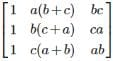, then Det. A is

Detailed Solution for Test: Determinants - 1 - Question 1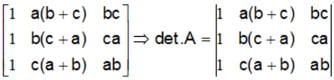Apply C2 → C2 + C3,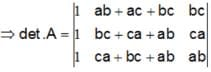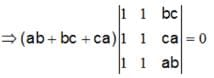Test: Determinants - 1 - Question 2

###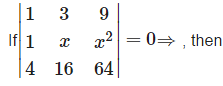Detailed Solution for Test: Determinants - 1 - Question 2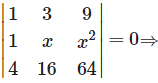because , the value of the determinant is zero only when , the two of its rows are identical., Which is possible only when Either x = 3 or x = 4 .

Test: Determinants - 1 - Question 3

###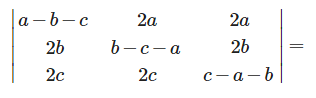Detailed Solution for Test: Determinants - 1 - Question 3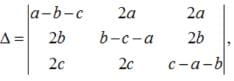Apply , R1 → R1+R2+R3,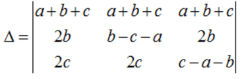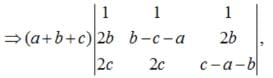Apply , C3→ C- C1, C2C2 - C1,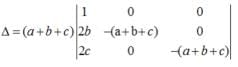=(a+b+c)3

Test: Determinants - 1 - Question 4

If A and B are invertible matrices of order 3 , then det (adj A) =

Detailed Solution for Test: Determinants - 1 - Question 4

Let A be a non singular square matrix of order n . then , |adj.A| = 1

Test: Determinants - 1 - Question 5

If A and B matrices are of same order and A + B = B + A, this law is known as

Detailed Solution for Test: Determinants - 1 - Question 5

Commutative law, in mathematics, either of two laws relating to number operations of addition and multiplication, stated symbolically: a + b = b + a and ab = ba

Test: Determinants - 1 - Question 6

If A’ is the transpose of a square matrix A , then

Detailed Solution for Test: Determinants - 1 - Question 6

The determinant of a matrix A and its transpose always same.

Test: Determinants - 1 - Question 7

The value of the determinant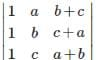is

Detailed Solution for Test: Determinants - 1 - Question 7, Apply , C2 → C2 + C3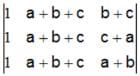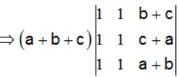= 0, (∵ C1 = C2)

Test: Determinants - 1 - Question 8

The roots of the equation det.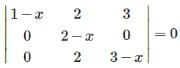are

Detailed Solution for Test: Determinants - 1 - Question 8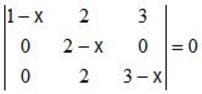⇒ (1-x)(2-x)(3-x) = 0 ⇒x = 1,2,3

Test: Determinants - 1 - Question 9

If A is a square matrix of order 2 , then det (adj A) = x

Detailed Solution for Test: Determinants - 1 - Question 9

Let A be a square matrix of order 2 . then ,|adj.A| = |A|

Test: Determinants - 1 - Question 10

If A is a symmetric matrix, then At =

Test: Determinants - 1 - Question 11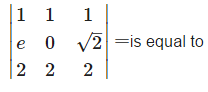Detailed Solution for Test: Determinants - 1 - Question 11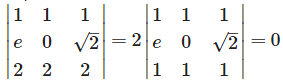, because , row 1 and row 3 are identical.

Test: Determinants - 1 - Question 12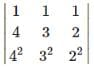is equal to

Detailed Solution for Test: Determinants - 1 - Question 12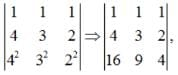Apply , C1→C1 - C3, C2→C2-C3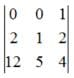= 10 - 12 = -2

Test: Determinants - 1 - Question 13

If A+B+C = π, then the value of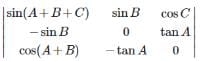Detailed Solution for Test: Determinants - 1 - Question 13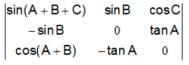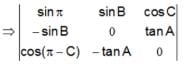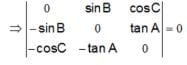Test: Determinants - 1 - Question 14

If A is a non singular matrix of order 3 , then |adj(A3)| =

Detailed Solution for Test: Determinants - 1 - Question 14

If A is anon singular matrix of order , then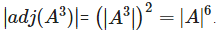Test: Determinants - 1 - Question 15

If A and B are any 2 × 2 matrices , then det. (A+B) = 0 implies

Detailed Solution for Test: Determinants - 1 - Question 15

Det.(A+B) ≠ Det.A + Det.B.

Test: Determinants - 1 - Question 16

If A B be two square matrices such that AB = O, then

Detailed Solution for Test: Determinants - 1 - Question 16

If A B be two square matrices such that AB = O, then,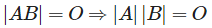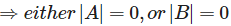Test: Determinants - 1 - Question 17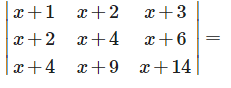Detailed Solution for Test: Determinants - 1 - Question 17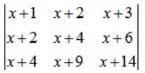Apply , C1 → C1 - C2, C2 → C2 - C3,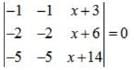Because here row 1 and 2 are identical

Test: Determinants - 1 - Question 18

If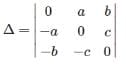, then equals

Detailed Solution for Test: Determinants - 1 - Question 18

Because , the determinant of a skew symmetric matrix of odd order is always zero and of even order is a non zero perfect square.

Test: Determinants - 1 - Question 19

If I3 is the identity matrix of order 3 , then 13−1 is

Detailed Solution for Test: Determinants - 1 - Question 19

Because , the inverse of an identity matrix is an identity matrix.

Test: Determinants - 1 - Question 20

If A and B are square matrices of same order and A’ denotes the transpose of A , then

Detailed Solution for Test: Determinants - 1 - Question 20

By the property of transpose of a matrix ,(AB)’ = B’A’.

Test: Determinants - 1 - Question 21

A square matrix A is invertible iff det A is equal to

Detailed Solution for Test: Determinants - 1 - Question 21

Only non-singular matrices possess inverse.

Test: Determinants - 1 - Question 22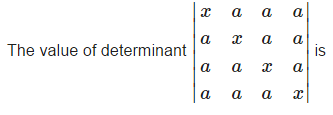Detailed Solution for Test: Determinants - 1 - Question 22Apply, C1→ C1+ C2+C3+C4,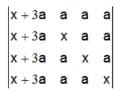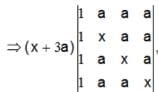Apply, R1 →R1 - R2,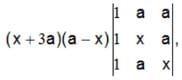Apply, R1 R- R2, R→ R- R3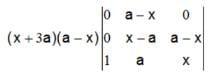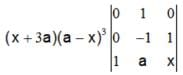=(x+3a) (a -x)3 (1) = (x+3a)(a-x)3

Test: Determinants - 1 - Question 23

If the entries in a 3 x 3 determinant are either 0 or 1 , then the greatest value of this determinant is :

Detailed Solution for Test: Determinants - 1 - Question 23

Greatest value = 2

Test: Determinants - 1 - Question 24

The roots of the equation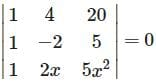are

Detailed Solution for Test: Determinants - 1 - Question 24

Operate,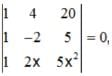Apply R3→R3- R1, R2 R2 -R1,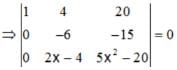⇒ -6(5x2 - 20) +15(2x-4) = 0

⇒ (x- 2)(x+1) = 0⇒x=2, -1

⇒-6(5x2 - 20) + 15(2x-4) = 0

⇒(x-2)(x+1) = 0 ⇒ x=2, -1

Test: Determinants - 1 - Question 25

In a third order determinant, each element of the first column consists of sum of two terms, each element of the second column consists of sum of three terms and each element of the third column consists of sum of four terms. Then it can be decomposed into n determinants, where n has value

Detailed Solution for Test: Determinants - 1 - Question 25

N = 2 ×3 × 4 = 24.

## Mathematics (Maths) Class 12

209 videos|218 docs|139 tests
Information about Test: Determinants - 1 Page
In this test you can find the Exam questions for Test: Determinants - 1 solved & explained in the simplest way possible. Besides giving Questions and answers for Test: Determinants - 1, EduRev gives you an ample number of Online tests for practice

## Mathematics (Maths) Class 12

209 videos|218 docs|139 tests(Scan QR code)